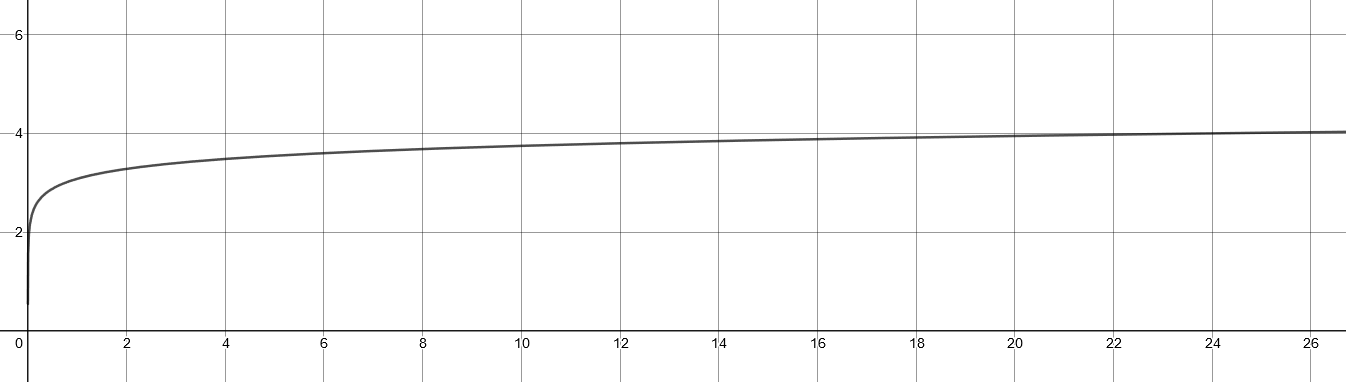# W19 Assignment 6

Updated 2019-11-07

See the attached pages at the back of the submission for hand-written work.

## Hydrostatic Equilibrium

A certain planet has density $\rho(r)$ that decreases linearly going from the core to the surface, so that $\rho(r)=\rho_0(1-2r/3R)$, where $r$ is the radial coordinate and $R$ is the radius of the planet.

(a) derive an expression for the mass $M_r$ contained within radius $r$.

The density function describes the density of a particular thin shell with radius $x$. The infinitesimal volume of the thin shell is:

$\mathop{dV}=4\pi x^2 \mathop{dx}$

The mass for these shell is then:

\begin{aligned} m(x)&=\rho(x)\mathop{dV}\\ &=\rho_0\left(1-\frac{2x}{3R}\right)\cdot 4\pi x^2 \mathop{dx} \end{aligned}

Then we can integrate from x = 0 → r to get the mass inside the radius $r$.

\begin{aligned} M_r&=\frac{4\pi\rho_0}{3R}\int_0^r(1-2x)x^2\mathop{dx}\\ &=\frac{4\pi\rho_0}{3R}\int_0^r x^2-2x^3\mathop{dx}\\ &=\frac{4\pi\rho_0}{3R} \left[ \frac{1}{3}x^3-\frac{1}{2}x^4 \right]^r_0\\ &=\frac{4\pi\rho_0}{3R} \left( \frac{1}{3}r^3-\frac{1}{2}r^4 \right)\\ \end{aligned} \begin{aligned} M_r&=4\pi\rho_0\int_0^r\left(1-\frac{2x}{3R}\right)x^2\mathop{dx}\\ &=4\pi\rho_0\int_0^rx^2-\frac{2x^3}{3R}\mathop{dx}\\ &=4\pi\rho_0\left[\frac{x^3}{3}-\frac{x^4}{6R}\right]^r_0\\ &=4\pi\rho_0\left(\frac{r^3}{3}-\frac{r^4}{6R}\right) \end{aligned}

(b) Derive dP/dr.

Start by considering an imaginary cylinder with mass $dm$ and height of $dr$ radially from the center of the sphere. Then start from Newton’s law of force:

$F=ma$

Where forces are the forces from top pressure and bottom pressure of the cylinder acting on the cylindrical mass. And acceleration is just double-derivative of the radial position: $\ddot r$. Formalizing the equation:

$(dm)(\ddot r) = - F_g + F_{P_B} - F_{P_T}$

Where

$F_g = \frac{G M_r dm}{r^2} \simeq \frac{G M_r}{r^2}$

And since we’re considering hydrostatic equilibrium, the acceleration should be 0: $\ddot r = 0$.

Putting it all together, and cancelling $F_{P_B} - F_{P_T}$ into $A dP$, we get:

$\rho \ddot r = \frac{G M_r \rho}{r^2}-\frac{dP}{dr},\quad\ddot r = 0$

Isolate for dP/dr, and substitute $M_r$ for the expression from part (a), and substitute the expression for $\rho=\rho(r)$:

$\frac{dP}{dr}=\frac{G}{r^2}\times 4\pi \rho\left(\frac{r^3}{3}-\frac{r^4}{6R}\right)\times \rho_0 \left(1-\frac{2r}{3R}\right)$

Simplifying the above equation and we get a three-term polynomial:

$\frac{dP}{dr}=4\pi\rho_0 ^2G\left(\frac{r}{3}-\frac{7 r^2}{18 R} + \frac{2r^3}{18 R^2}\right)$

(c) To get pressure, we integrate.

\begin{aligned} P(r)&=\int{\frac{dP}{dr}\mathop{dr}}\\ &=\int{4\pi\rho_0 ^2G\left(\frac{r}{3}-\frac{7 r^2}{18 R} + \frac{2r^3}{18 R^2}\right)\mathop{dr}}\\ &=4\pi\rho_0 ^2G\int{\left(\frac{r}{3}-\frac{7 r^2}{18 R} + \frac{2r^3}{18 R^2}\right)}\mathop{dr}\\ &=4\pi\rho_0 ^2G\times\left(\frac{1}{6}r^2-\frac{7}{54R}r^3+\frac{1}{36R^2}r^4+C\right) \end{aligned}

To find C, we set $r=R$, and assume that the pressure at surface $P(R)=0$:

\begin{aligned} P(R)=0&=\left(\frac{1}{6}r^2-\frac{7}{54R}r^3+\frac{1}{36R^2}r^4+C\right)\\ 0&=\frac{7R^2}{108}+C\\ C&=-\frac{7R^2}{108} \end{aligned}

Putting it all together:

$P(R)=4\pi\rho_0^2 G \left(\frac{1}{6}r^2-\frac{7}{54R}r^3+\frac{1}{36R^2}r^4-\frac{7R^2}{108}\right)$

## Degeneracy Pressure

(a) Find degeneracy pressure in number density.

Assuming that the star is full of H2 gas with uniform density. The mass of the hydrogen atom is 1.67×10-27 kg. So that means 1kg of Hydrogen has 5.988×1026 atoms. This is our number density for the electrons since a hydrogen atom only has one electron.

Then for a given mass density $\rho$ in the units kg/m3, we have $\rho\times5.988\times10^{26}$ number density in the units e-/m3.

Then we plug into the equation given from class:

$P=\frac{1}{20}\left(\frac{3}{\pi}\right)^{2/3}\frac{h^2}{m_e}n_e^{5/3}\\ P=\frac{1}{20}\left(\frac{3}{\pi}\right)^{2/3}\frac{h^2}{m_e}(\rho\times 5.988\times 10^{26})^{5/3}$

(b) Equation of boundary between non-degeneracy and degeneracy.

Starting from

$P_{termal}=\frac{\rho}{m_p}k_BT$

Then we plug in the expressions from part (a), then isolate for T:

\begin{aligned} T&= \frac{P_{thermal} m_p}{\rho k_B}\\ &= \frac{\left(\frac{1}{20}\left(\frac{3}{\pi}\right)^{2/3}\frac{h^2}{m_e}(\rho\times 5.988\times 10^{26})^{5/3}\right) m_p}{\rho k_B}\\ &=\left(\frac{3\rho}{\pi}\right)^{2/3}\frac{h^2(5.988\times10^{26})^{5/3}m_p}{20m_ek_B}\\ \end{aligned}

The entire rightmost side can be evaluated down to a constant:

$\frac{h^2(5.988\times10^{26})^{5/3}m_p}{20m_ek_B}=1242$

So therefore:

$T=1242\left(\frac{3\rho}{\pi}\right)^{2/3}$

(c) Plot

Here is a log-log plot of the above function:Where the y-axis is log10(T) and x-axis is log10(ρ). The region of degeneracy is above the graph.

(d) Saturn

For a mass density of 3000 kg/m3, we plug it into the equation we derived in (b):

$T_D=\left(\frac{3(3000)}{\pi}\right)^{2/3}1242=251\times 10^3K$

The actual temperature is $\sim 40,000K$, so there is no degeneracy. So it lies in part of the graph that is below the boundary curve.

## Neutron-Star Breakup Speed

(a) Find maximum orbit frequency

We can assume that surface mass is attached to the neutron star if it’s not orbiting. Then we can start by calculating the required circular orbit speed at the surface:

$v_c=\sqrt{\frac{G M}{r}}=\sqrt{\frac{G (1.4M_\odot)}{10,000}}$

Plugging in the constants, we get a orbit speed of $v_c=1.36\times 10^8$ m/s which is very fast.

The equatorial circumference of the neutron star is $2\pi(10\text{km})$, and therefore the period is distance divided by speed:

$P=\frac{2\pi 10,000}{1.36\times 10^8}=0.4609\times 10^{-3} \text{s}$

(b) equatorial bulge

The relationship between the polar and equatorial radius is given by:

$\frac{E-P}{R}=\frac{5\Omega^2R^3}{4GM}$

So we plug everything in where $\Omega$ is the angular frequency defined by:

$\Omega = 2\pi P^{-1}$

Then

$E-P = \frac{5\Omega^2 R^4}{4G (1.4 M_\odot)}=1.374 \text{km}$

To solve for E and P, we can also assume that the volume of the ellipsoid and the sphere don’t change:

$V_{sphere}=V_{ellipsoid}\\ r^3=E^2 P, \quad r=10\text{km}, \quad P=E-1.374\text{km}$

We have two equations and two unknowns, so we solve for both:

$E = 10.48\text{km},\quad P=9.106\text{km}$